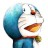# 杰拉斯的博客

## [ACM_NYOJ_17]单调递增最长子序列杰拉斯 | 时间：2013-05-04, Sat | 7,485 views

### 单调递增最长子序列

```3
aaa
ababc
abklmncdefg
```

```1
3
7
```

#### 来源

NYOJ17

```#include<iostream>
#include<string>

using namespace std;

int main(){
int t;
string s;
cin >> t;
while(t--){
cin >> s;
int ans = 0, f;
for(int i = 0, len = s.length(); i < len; ++i){
int max = 0;
for(int j = 0; j < i; ++j){
if(s[j] < s[i] && max < f[j]){
max = f[j];
}
}
if((f[i] = max + 1) > ans){
ans = f[i];
}
}
cout << ans << "\n";
}
return 0;
}
```